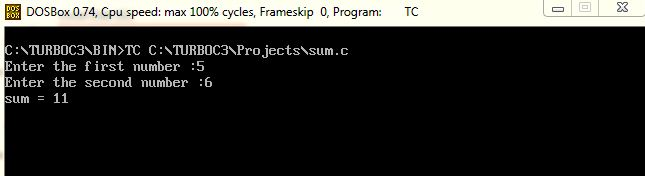UncleCoder.com

UncleCoder.com

Free programming examples and instructions

# C Program to add two numbers

## C program for add any two numbers

by Krishna

Posted on 27 Jul 2018 Category: C Views: 2297

C Program to Add any two numbers

We will write a program to find the sum of two integer numbers entered by user. In this program, the user is asked to enter two integer numbers and then the program displays the sum of these numbers. To read the input numbers we are using scanf() function and then we are using printf() function to display the sum of these numbers.

PROGRAM:

``````#include <stdio.h>
int main()
{
int num1, num2, sum;
printf("Enter the first number: ");
scanf("%d", &num1);
printf("Enter the second number: ");
scanf("%d", &num2);
sum = num1 + num2;
printf("sum = %d", sum);
return 0;
}``````

OUTPUTRelated Article

C program to find sum of first 10 integers using for loop

C program to find largest among two numbers

C Program to find sum of two numbers using Function

C Program to find sum of digits

C Program to swap two numbers using Pointers

C program to find the factors of a number

C program to find Reverse of a Number

C program to swap two numbers without using third variable